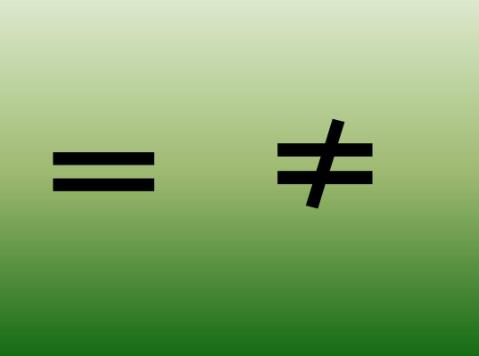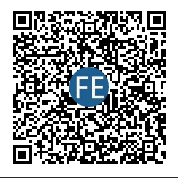# js如何判断两个对象数组是否相等？

``````let arr1 = [{a: 1}, {b: 2}]
let arr2 = [{a: 1}, {b: 2}]
``````

1、完全相等；（包含顺序相同）
2、仅元素相等；（顺序不一定相同）

### 判断两个数组完全相等的方法：

``````function isEqualArray(arr1, arr2){
return Array.isArray(arr1) &&
Array.isArray(arr2) &&
arr1.length === arr2.length &&
JSON.stringify(arr1) === JSON.stringify(arr2)
}
``````

### 判断两个数组仅元素相等的方法：

###### 第四步：如果元素的属性值是一个Function、Date、Symbol、Array呢，又要怎么判断

……### 首先，判断数组元素的是否相等，先要判断元素是否一个对象：

``````function isPlainObject(obj){
return typeof obj === 'object' && Object.prototype.toString.call(obj) === '[object Object]'
}
``````

### 然后判断这个对象内容是否相等，即属性是否相等：

``````function isEqualObject(obj1, obj2){
if( !isPlainObject(obj1) || !isPlainObject(obj2)  ){
return obj1 === obj2
}
if(Object.keys(obj1).length !== Object.keys(obj2).length){
return false
}
for(let key in obj1){
if( isPlainObject(obj1[key]) && isPlainObject(obj2[key])  ){
if(!isEqualObject(obj1[key], obj2[key])){
return false
}
}else if(obj1[key] !== obj2[key]){
return false
}
}
return true
}
``````

### 这样的话，判断两个对象数组仅元素相等的方法就出来了：

``````function isEqualArray(arr1, arr2){
if( !Array.isArray(arr1) || !Array.isArray(arr2)){
return false
}

if(arr.length !== arr2.length){
return false
}

for ( let i = 0; i < arr1.length; i++) {
if(isPlainObject(arr1[i]) && isPlainObject(arr2[i])){
if( !isEqualObject(arr1[i], arr2[i])){
return false
}
}else if(arr1[i] !== arr2[i]){
return false
}
}
return true
}
``````734802480（已满）、 794324979

﻿